# Below is the p.d.f for the random variables X and Y, f(x,y)-36 0 otherwise Find the...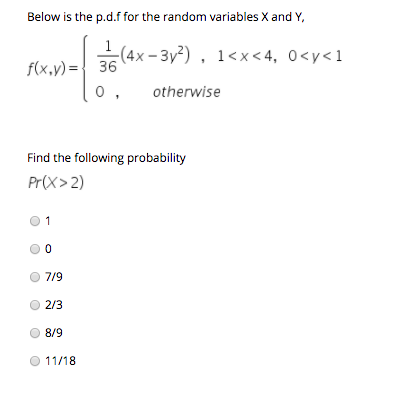Below is the p.d.f for the random variables X and Y, f(x,y)-36 0 otherwise Find the following probability Pr(x> 2) O 7/9 2/3 O 8/9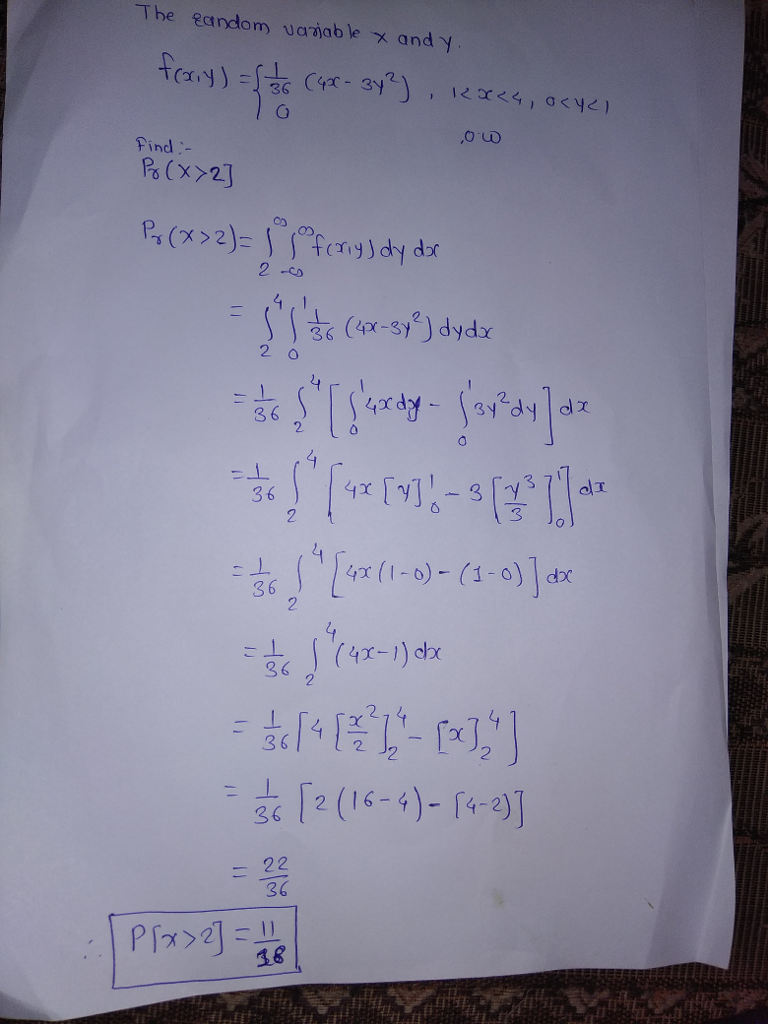#### Earn Coin

Coins can be redeemed for fabulous gifts.

Similar Homework Help Questions
• ### Find Var（2X-Y） Two random variables X and Y are i.i.d. and their common p.d.f. is given...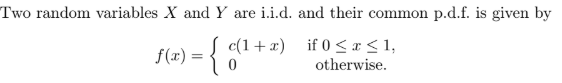Find Var（2X-Y） Two random variables X and Y are i.i.d. and their common p.d.f. is given by f )- c(1+r) if 0 <r < 1. otherwise. f(3) = 10

• ### 2. If X and Y are independent random variables, X has a normal distribution with mean...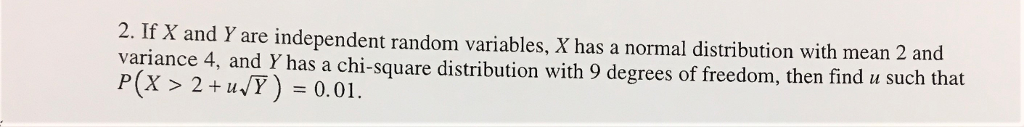2. If X and Y are independent random variables, X has a normal distribution with mean 2 variance 4, and Y has a chi-square distribution with 9 degrees of freedom, then find u such that P(X > 2+11,7)=0.01.

• ### 3. Let {X1, X2, X3, X4} be independent, identically distributed random variables with p.d.f. f(0) =...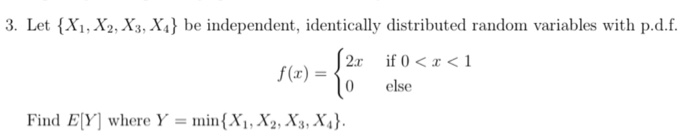3. Let {X1, X2, X3, X4} be independent, identically distributed random variables with p.d.f. f(0) = 2. o if 0<x< 1 else Find EY] where Y = min{X1, X2, X3, X4}.

• ### (6 points) Let X and Y be independent random variables with p.d.f.s fx(x) -{ { 1-22...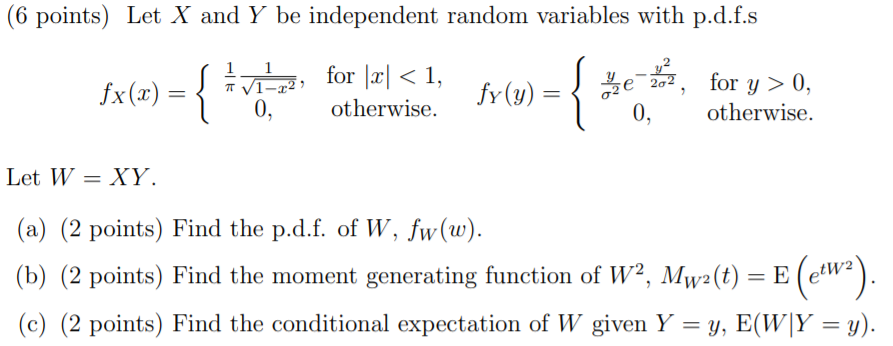(6 points) Let X and Y be independent random variables with p.d.f.s fx(x) -{ { 1-22 0, for |2|<1, otherwise. fy(y) = for y>0, otherwise. 0, Let W = XY (a) (2 points) Find the p.d.f. of W, fw(w). (b) (2 points) Find the moment generating function of W2, Mw?(t) = E (e«w?). (c) (2 points) Find the conditional expectation of W given Y = y, E(W|Y = y).

• ### 6. Suppose that X and Y are jointly continuous random variables with joint density f(r, y)otherwise...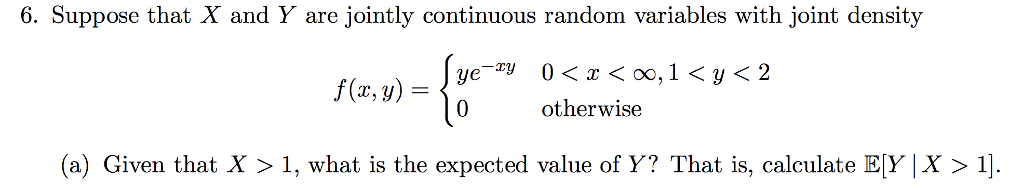6. Suppose that X and Y are jointly continuous random variables with joint density f(r, y)otherwise (a) Given that X > 1, what is the expected value of Y? That is, calculate Ey X 〉 1).

• ### Random variables z and y described by the PDF if x-+ yo 1 and x.> 0...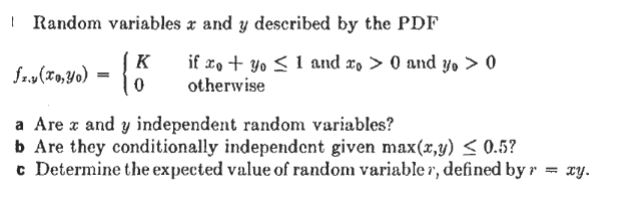Random variables z and y described by the PDF if x-+ yo 1 and x.> 0 and y, > 0 0 otherwise a Are x and y independent random variables? b Are they conditionally independent given max(x,y) S 0.5? c Determine the expected value of random variabler, defined byr xy.

• ### A continuous random variable X has a beta distribution with p.d.f : 1 f(x) = 0<<<1,...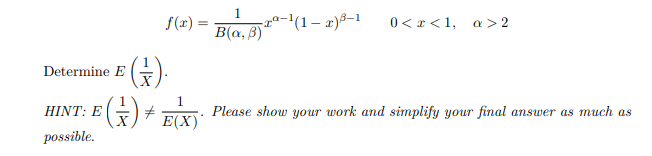A continuous random variable X has a beta distribution with p.d.f : 1 f(x) = 0<<<1, a > 2 B(4, 5)22-1(1 – 2)8-1 Determine E (3) HINT: E possible. (-) + E(X) Please show your work and simplify your final answer as much as

• ### 5. (50pt) X and Y are continuous random variables with pdf f(x, y) 2r for 0...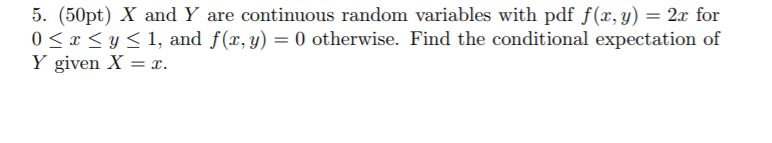5. (50pt) X and Y are continuous random variables with pdf f(x, y) 2r for 0 < x y < 1, and f(x,y) = 0 otherwise. Find the conditional expectation of Y given X = z.

• ### 1) Let random variables X and Y have the joint PMF: otherwise a) Calculate the value...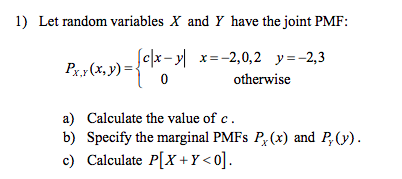1) Let random variables X and Y have the joint PMF: otherwise a) Calculate the value of c b) Specify the marginal PMFs Pr(x) and P- c) Calculate P[X +Y<0].

• ### Exercise 5. The joint probability density function of X and Y is given by (X,Y)=9) Scy-re-y...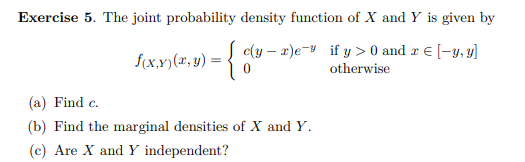Exercise 5. The joint probability density function of X and Y is given by (X,Y)=9) Scy-re-y if y> 0 and -y, y) O otherwise (a) Find c. (b) Find the marginal densities of X and Y. (c) Are X and Y independent?